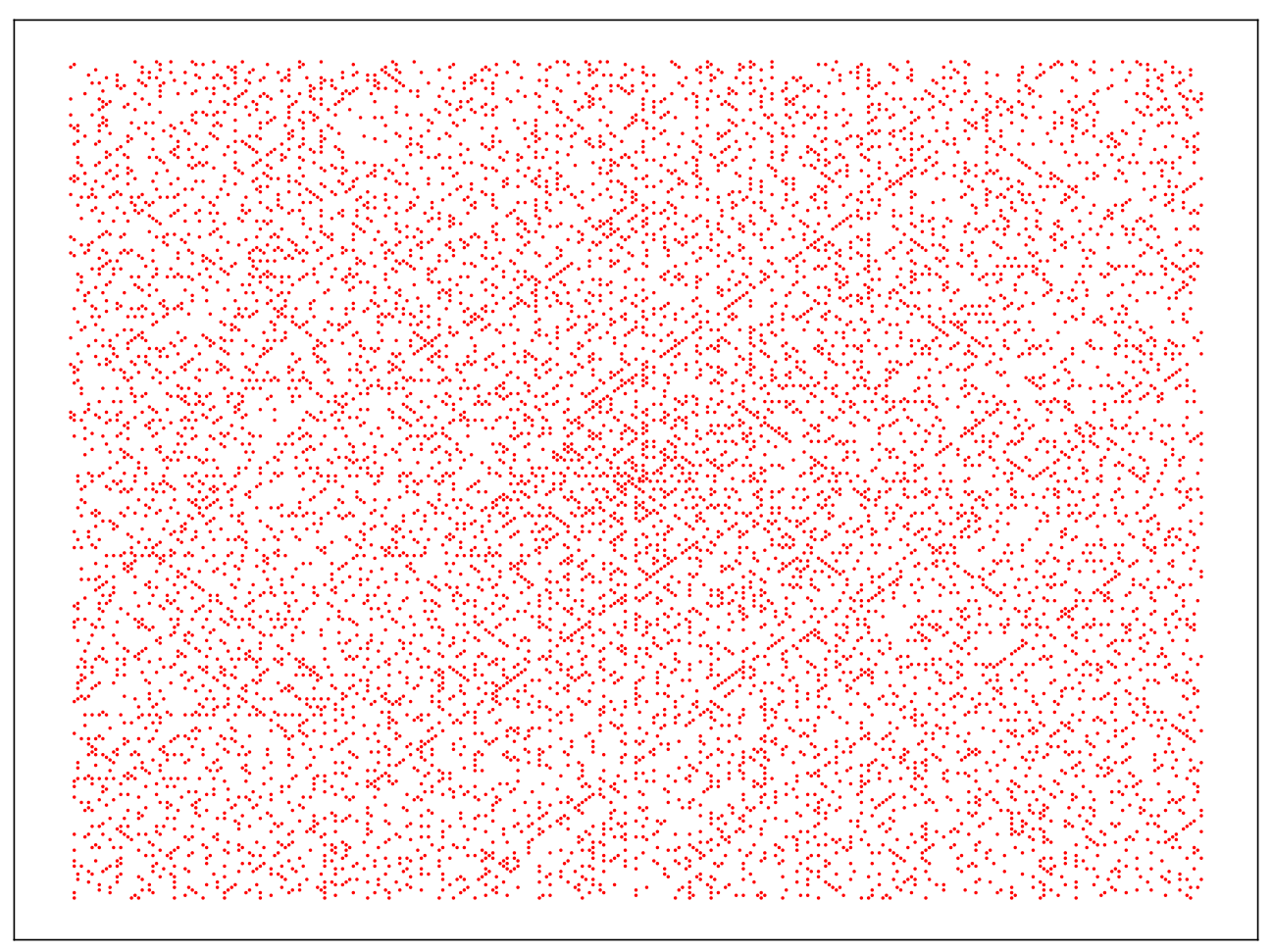Ever since I was in school, I had this werid habit of adding the digits of number around me. Looking at number plates of the cars driving around me, or the numbers flashing on the cable television channels occasionally. By the way the flashing numbers are an anti-piracy measure so that if someone records a TV stream they can track it to the person who did. Things you learn every day.

This blog post is just me playing with digit sums and concept of digit root and identifying some cool number theoretic observations.

## Digit Sums

I was wondering a while back what interesting patterns might emerge by adding digits of numbers repeatedly untill I get a single digit number. I quickly realised though to my dismay that the answer was pretty obvious. Each set of 10 digits will always give the sum back as 1 to 9. Try it with 51 to 59 yourself, 51 will add upto 6, 52 will add upto 7 and as we move ahead we get to 55, which gives us the sum as 10 which in turn gets added up to 1 followed by 2 , 3, 4 and 5 for 59.

Therefore, if we add digits for all the numbers and look at the number of times it appears we should get a uniform distribution of each digit.

This kind of repeated addition is known as digit root ($$S_r(k)$$) in number theory. And if we only do it once we call it a digit sum ($$S(k)$$).

i.e. $$S(56)=11$$ and $$S_r(56)=2$$.

In julia we get a predefined function which gives us the digits in an array

digits()

using CairoMakie,StatsBase,PlutoUI
digits(100) #shows all digits as an array
3-element Vector{Int64}:
0
0
1

We can quickly write a primitive code that collects all sums of each digits from 1 to 10 million. And we can then count the number of times they appear

Dict{Any, Int64} with 9 entries:
5 => 1111111
4 => 1111111
6 => 1111111
7 => 1111111
2 => 1111111
9 => 1111111
8 => 1111111
3 => 1111111
1 => 1111111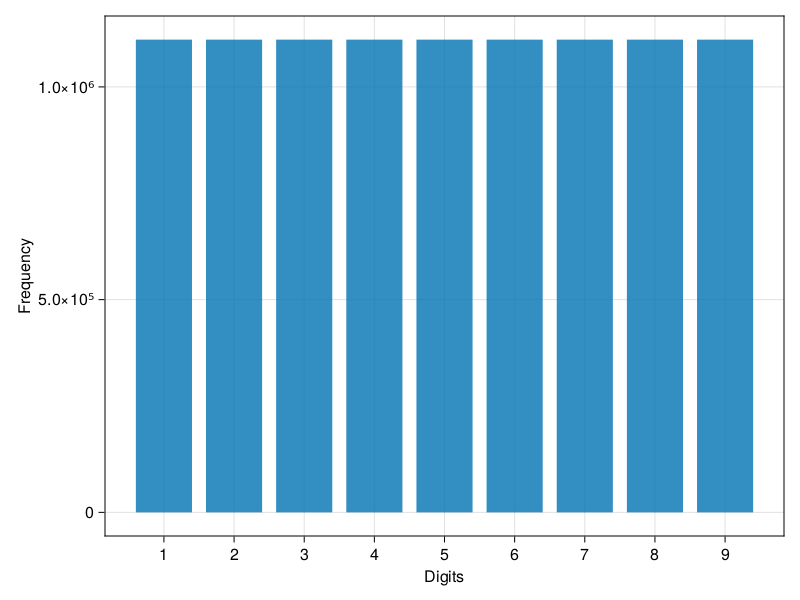As one can clearly see the distribution is uniform and there are $$1,111,111$$ appearance of each digit in counting iterative digit sum of all numbers beginning from 1 to $$10^6-1$$

This was certainly a little interesting but very obvious. How can we make it more interesting?

## Prime Numbers

It is our number system making sure that 1 to 9 digits in our decimal system is counted once that we get this nice and uniform distribution. What if we seldct them randomly? We will definitely not get a perfect uniform distribution. This led me to thinking about something more fun. What if we look at all prime numbers?

Certainly prime numbers are not uniformlly distributed and distribution of prime numbers do show some very cool patterns. And has been a subject for research.

See Ulam Spiral and Prime Number Theorem (PNT)

For this we will use Primes.jl library in Julia

using Primes #Importing the library
plist=Primes.primes(10^8) #Listing all prime numbers till 10^8
5761455-element Vector{Int64}:
2
3
5
7
11
13
17
⋮
99999847
99999931
99999941
99999959
99999971
99999989

Let us perform the same iterative sum method on the set of prime numbers that we now have.

cmap1=StatsBase.countmap(SUMS1)
Dict{Any, Int64} with 7 entries:
5 => 960278
4 => 960360
7 => 960198
2 => 960341
8 => 960318
3 => 1
1 => 959959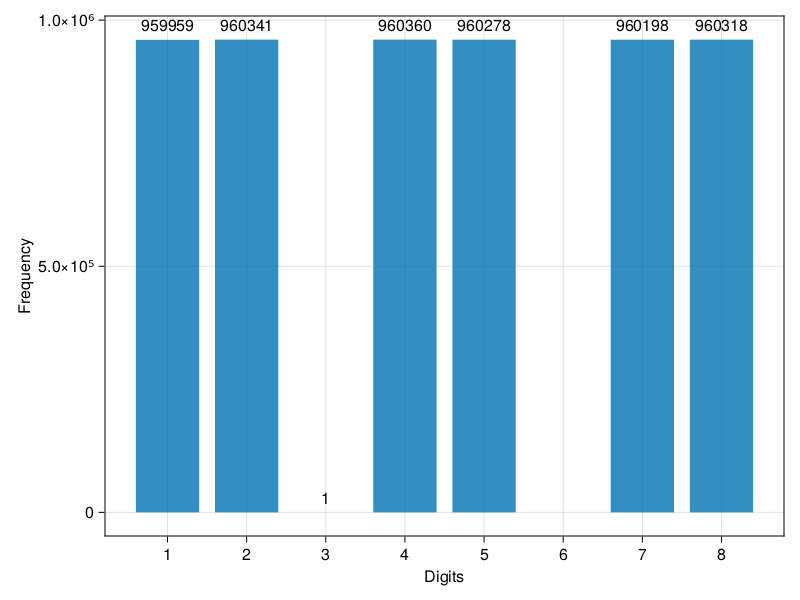Immediately we notice that 6 and 9 are missing from the distribution. And 3 only appears once as itself. So, why are there no prime numbers(>3) that iteratively gives out sum as 3,6 and 9?

This is an obvious thing for a number thoerist. But not so obvious for me. I found out quickly on the internet that it is connected to the divisible test that we learnet in school as mental maths. If the sum of the digits of a number is divisble by 9 the number is also divisible by 9. And if it is divisible by 9, it is also divisible by 3. Therefore by definition all prime numbers who are not divisible by 9 do not appear in this category.

Instead of looking at the iterative sum untill we reach a single digit number, we could just consider the sum of the digits once and then look at the distribution.

begin
SUMS2=[]
for x in plist
#println(x,"\t",sum(digits(x)))
S=sum(digits(x))
append!(SUMS2,S)
end
end
get_SUMS (generic function with 1 method)
get_SUMS_prime (generic function with 1 method)
CairoMakie.hist(Int32.(SUMS2))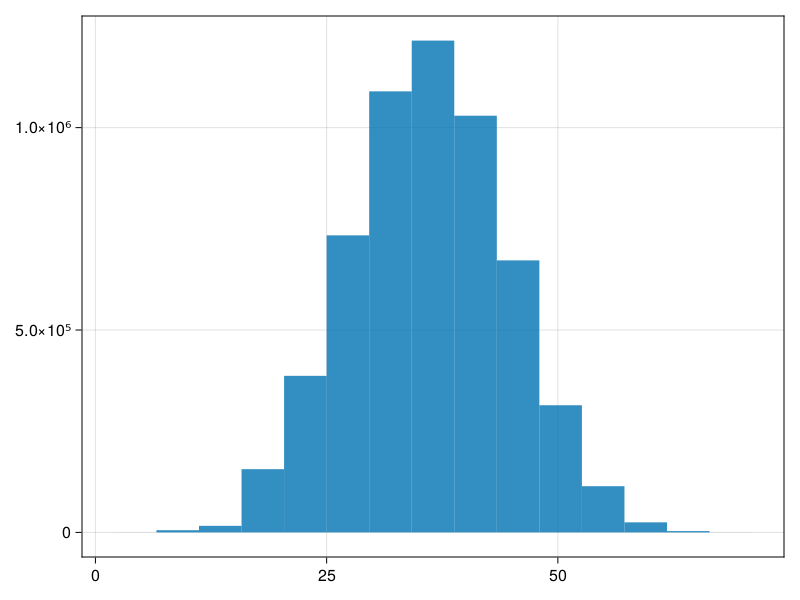mean(SUMS2),√var(SUMS2)
(36.31324535208554, 8.259968573266114)

When we do that the Good Old Normal distribution returns. We see that the mean of the digit sum for all prime numbers upto 10^8 is $$\mu\simeq 36$$ with $$\sigma\simeq8$$

This digit sum and digit root is not just number theoretic fun. As we can see from this post about a paper: a group of inter disciplanry researchers have found a connection between digit sums and a fractal curve related to digit sum with the genetics. The robustness of phenotype expression due to a point mutation in the genotype of an organism is bound by a curve whose closed form comes from the digit sum. A very interesting research paper that shows how the abstract number theory and mathematics can be applied in other scientific field if we open our minds to it and think outside the box. So, what about fractals in digit sum functions? One of the obvious simple fractal we see is explored below.

## Fractals in Digit sums

If we plot all the digit sums with the number in the x-axis and its sum in the y-axis we see a crazier result. We see a repeating chain like pattern that repeats 10 times. 10 ofcourse makes sense as we are working in the Base 10 Decimal system.

### For Prime Numbers

Notice the gaps in the sums, the gaps correspond to those numbers whose sum gets added to numbers like 18, 21, 45, etc which then gives the digit root as 3,6 or 9.

CairoMakie.scatter(plist,Int32.(SUMS2),markersize=8,color=(:black,0.5))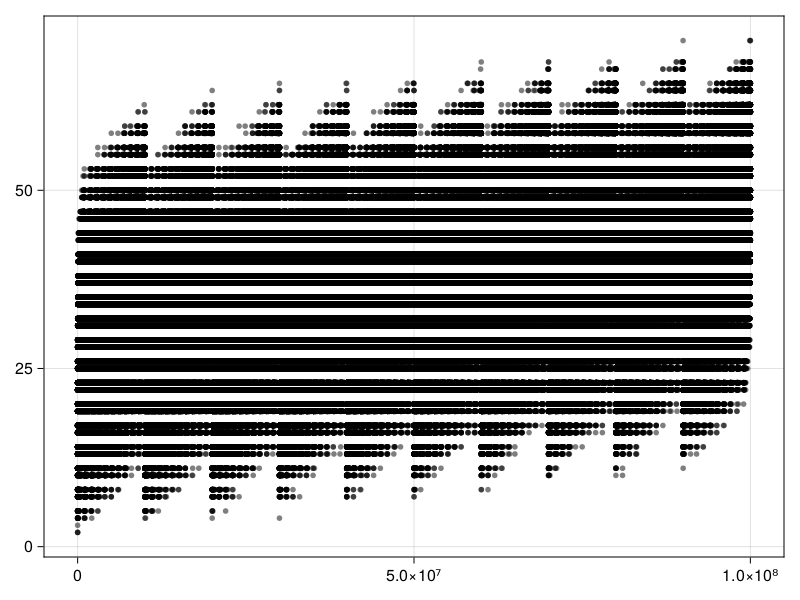It gets more interesting when we do the same process at various scales. Below you can see similar self repeating pattern at different scales (10^3,10^4,10^6 and 10^8). If we look at the numbers and their sum in Base 4, we get 4 zig-zag repeating chains.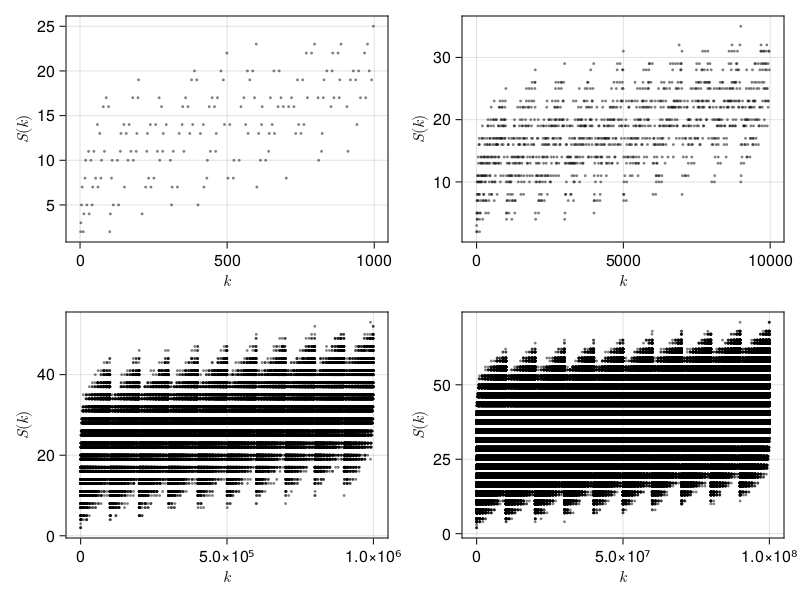### For all numbers

Since this fractal behaviour appears simply because of the Base of the number system you are using same behaviour can be seen if we perform the digit sum of all integers Following graph shows fractal behaviour for all numbers at the same scales

begin
figcollage1=CairoMakie.Figure()
itt=1
#	axs=[[1,1],[1,2],[2,1],[2,2]]
for pmax in [10^3,10^4,10^5,10^6]
plist,SUMS0=get_SUMS(1,pmax)
axcollage=CairoMakie.Axis(figcollage1[axs[itt],axs[itt]], xlabel=L"k",ylabel=L"S(k)")
if itt>2
markersize=2
else
markersize=4
end
CairoMakie.scatter!(axcollage,plist,Int32.(SUMS0),markersize=markersize,color=(:black,0.4))
global itt=itt+1
end
figcollage1
end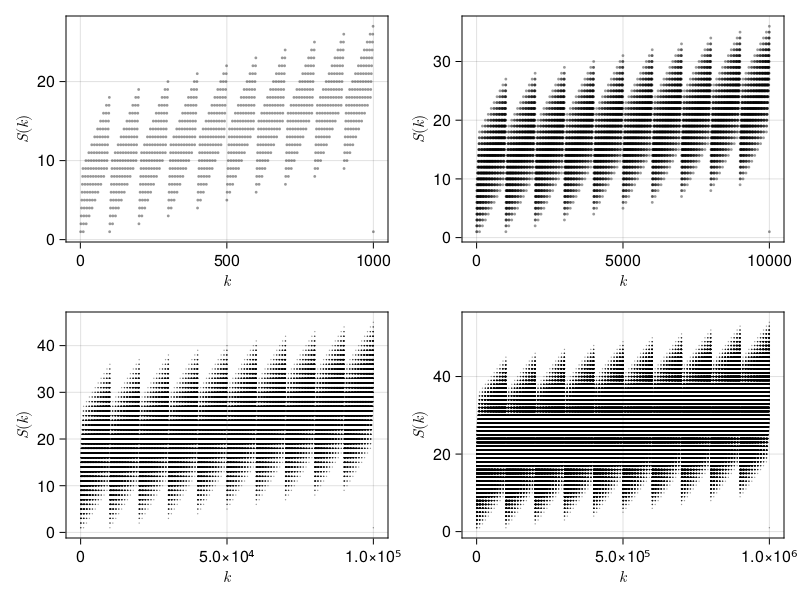# Bonus

## Ulam Spirals

As mentioned in the first section, Prime numbers show very cool pattern if highlighted in a square spiral. You start with 1 on the origin and then go around the origin turning by 90 degrees anti clockwise each time. You notice prominent diagonals in the ulam spiral drawn for prime numbers upto $$10^4$$. I have written a code below inspired from UlamSpiral.jl to draw the spiral for custom domain. As the wikipedia page for Ulam Spiral show, there exist prime generating quanratic polynomials like:

$$n^2+n+41=0$$

this provides prime numbers for $$n<40$$. And in q square spiral the quadratic functions are represented as diagonal straight lines.

position (generic function with 2 methods)
begin
xpoints=[]
ypoints=[]
prx=[]
pry=[]
for i in 1:10^4
point=position(i,1)
if isprime(i)
append!(prx,point)
append!(pry,point)
end
end
xpoints=Int64.(xpoints)
ypoints=Int64.(ypoints)
prx=Int64.(prx)
pry=Int64.(pry)
fig3=CairoMakie.Figure()
ax3=CairoMakie.Axis(fig3[1,1])
#CairoMakie.hidespines!(ax3)
#CairoMakie.hidedecorations!(ax3)
#CairoMakie.scatter!(ax3,xpoints,ypoints,markersize=2,color=(:black,0.5))
CairoMakie.scatter!(ax3,prx,pry,markersize=5,color=(:red,1))
#	CairoMakie.save("UlamSpiral10e4.pdf",fig3)
fig3
end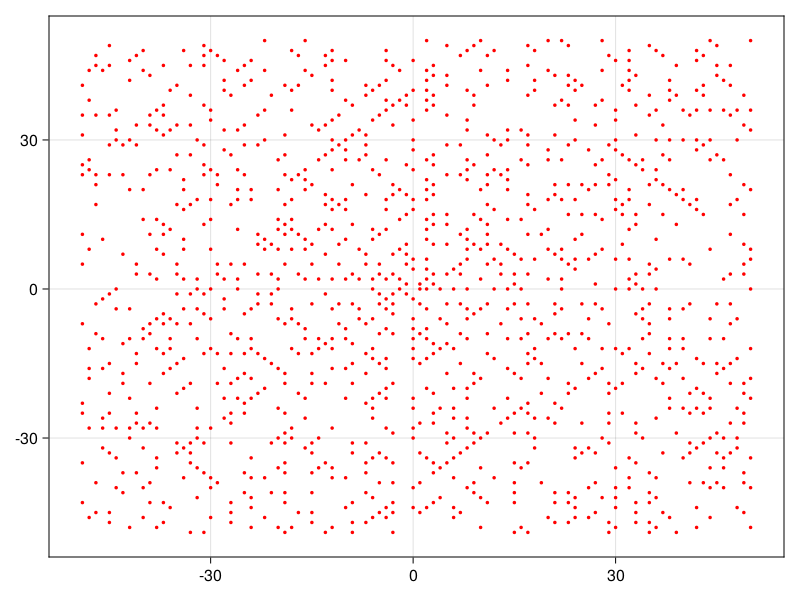Built with Julia 1.9.1 and

CairoMakie 0.10.8
PlutoUI 0.7.52
Primes 0.5.4
StatsBase 0.34.0
Ulam Spiral for prime numbers till $$10^5$$: